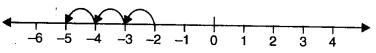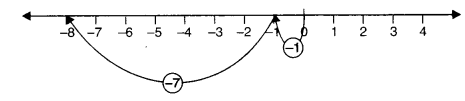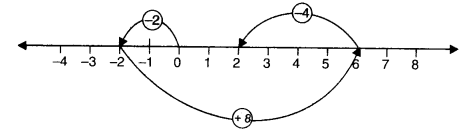NCERT Solutions for Class 6 Maths Chapter 6 Integers Ex 6.2 are part of NCERT Solutions for Class 6 Maths. Here we have given NCERT Solutions for Class 6 Maths Chapter 6 Integers Ex 6.2.

 Board CBSE Textbook NCERT Class Class 6 Subject Maths Chapter Chapter 6 Chapter Name Integers Exercise Ex 6.2 Number of Questions Solved 5 Category NCERT Solutions

## NCERT Solutions for Class 6 Maths Chapter 6 Integers Ex 6.2

Question 1.
Using number line write the integer which is:
(a) 3 more than 5
(b) 5 more than – 5
(c) 6 less than 2
(d) 3 less than – 2.
Solution.
(a) 3 more than 5
We start from 5 and proceed 3 steps to the right of 5 to reach 8 as shown below:Therefore, 3 more than 5 is 8.

(b) 5 more than – 5
We start from – 5 and move to the right by 5 steps and obtain 0 as shown below:Therefore, 5 more than – 5 is 0.

(c) 6 less than 2
We start from 2 and proceed 6 steps to the left of 2 to reach – 4 as shown below:(d) 3 less than – 2
We start from – 2 and move to the left by 3 steps and obtain – 5 as shown below:Therefore, 3 less than – 2 is – 5.

Question 2.
Use number line and add the following integers:
(a)
9 +(-6)
(b) 5+ (-11)
(c) (- 1) +(- 7)
(d) (-5)+ 10
(e) (-1) +(-2) + (-3)
(f) (-2) + 8 + (-4).
Solution.
(a) 9 +(-6)
On the number line, we first move 9 steps to the right from 0 reaching 9 and then we move 6 steps to the left of 9 and reach 3.
Thus, 9 + (- 6) = 3.(b) 5 + (-11)
On the number line, we first move 5 steps to the right from 0 reaching 5 and then we move 11 steps to the left of 5 and reach – 6.
Thus, 5 + (- 11) = – 6.(c) (-1) + (-7)
On the number line we first move 1 step to the left of 0 reaching – 1, then we move 7 steps to the left of – 1 and reach – 8.
Thus, (- 1) + (- 7) = – 8.(d) (- 5) + 10
First, we move 5 steps to the left of 0 reaching – 5, then from this point, we move 10 steps to the right. We reach the point + 5.
Thus, (- 5) + 10 = 5.(e) (-l) + (- 2) + (-3)
First, we move 1 step to the left of 0 reaching – 1, then from this point, we move 2 steps to the left to reach – 3 and finally from – 3, we move 3 steps to the left. We reach the point – 6.
Thus, (- 1) + (- 2) + (- 3) = – 6.(f) (- 2) + 8 + (- 4)
First, we move 2 steps to the left of 0 reaching – 2, then from this point, we move 8 steps to the right to reach + 6 and finally from + 6 we move 4 steps to the left. We reach the point 2.
Thus, (- 2) + 8 + (- 4) = 2.Question 3.
(a)
11 + (- 7)
(b) (- 13) + (+ 18)
(c) (-10) + (+ 19)
(d) (-250) + (+ 150)
(e) (- 380) + (- 270)
(f) (-217) + (-100).
Solution.
(a) 11 + (- 7)
11+(-7)
= 4 + 7 + (- 7)
=4+0=4

(b) (- 13) + (+ 18)
(-13)+ (+18)
= (- 13)+ (+.13) +(+5)
= 0 + (+5) = 5

(c) (-10) + (+ 19)
(-10)+ (+19)
= (- 10) + (+ 10) + (+ 9)
= 0 + (+ 9) = 9=

(d) (- 250) + (+ 150)
(- 250) + (+ 150)
= (- 100) + (- 150) + (+ 150)
= (- 100) + 0 = – 100

(e) (- 380) + (- 270)
(- 380) + (- 270)
= -650 (/) (- 217) + (-100)
= -317.

Question 4.
Find the sum of:
(a)
137 and – 354
(b) – 52 and 52
(c) – 312, 39 and 192
(d) – 50, – 200 and 300.
Solution.
(a) 137 and – 354
137+ (-354)
= 137+ (- 137)+ (-217)
= 0 +(-217) =-217

(b) – 52 and 52
– 52 + (52)
= 0

(c) – 312,39 and 192
(-312)+ (39)+ (192)
= (-312)+ (231)
= (-81)+ (-231)+ (231)
= (- 81) + 0 = – 81

(d) – 50, – 200 and 300
(-50)+ (-200)+ (300)
= (- 250) + (300)
= (- 250) + (250) + (50)
= 0 + (50) = 50.

Question 5.
Find the value of:
(a) (-7) +(-9)+ 4 + 16
(b) (37) +(-2) +(-65) +(-8).
Solution.
(a) (- 7) + (- 9) + 4 + 16
(- 7) + (- 9) + 4 + 16
= (-16)+ 20 = (-16) +16 + 4 =0+4=4

(b) (37) +(-2) +(-65) +(-8)
= (37)+ (75)
= (37)+ (-37)+ (-38) = 0 + (- 38)
= -38.

We hope the NCERT Solutions for Class 6 Maths Chapter 6 Integers Ex 6.2 help you. If you have any query regarding NCERT Solutions for Class 6 Maths Chapter 6 Integers Ex 6.2, drop a comment below and we will get back to you at the earliest.